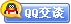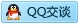##用户名 Email 自动登录 找回密码 密码 会员注册
 VIP会员，3年作业免费下 ！ 奥鹏作业，奥鹏毕业论文检测 新手作业下载教程，充值问题 没有找到答案，请在此处留言！ 2019年12月最新全国统考资料 投诉建议，加盟合作！奥鹏课程积分软件(ver:3.1)

# 12秋电子科大《C语言》在线作业2发表于 2012-10-12 10:33:15 | 显示全部楼层 |阅读模式谋学网: www.mouxue.com 主要提供奥鹏作业答案，奥鹏在线作业答案，奥鹏离线作业答案以及奥鹏毕业论文，致力打造中国最专业远程教育辅导社区。 一、单选题（共 35 道试题，共 70 分。）V 1.  以下正确的描述是( ) A. continue语句的作用是结束整个循环的执行 B. 只能在循环体内和switch语句体内使用break语句 C. 在循环体内使用break语句或continue语句的作用相同 D. 从多层循环嵌套中退出时, 只能使用goto语句       满分：2  分 2.  x,i,j和k都是int型变量,则执行下面表达式后x的值为( )(i=4,j=16,k=32) A. 4 B. 16 C. 32 D. 52       满分：2  分 3.  下面程序的运行结果是( ) #include "stdio.h" main() {int i; for(i=1;i<=5;i++) {if (i%2) printf("#"); else continue; printf("*"); } printf("\$"); } A. *#*#\$ B. #*#*#*\$ C. *#*#\$ D. #*#*\$       满分：2  分 4.  在C语言中,一维数组的定义方式为:类型说明符 数组名( ) A. [常量表达式] B. [整形表达式] C. [ 整型常量]或[整型表达式] D. [整型常量]       满分：2  分 5.  与下面程序段等价的是( ) for(n=100;n<=200; n++) {if (n%3==0) continue; printf("%4d",n);} A. for(n=100;(n%3)&& n<=200;n++) printf("%4d",n); B. for(n=100;(n%3)|| n<=200;n++) printf("%4d",n); C. for(n=100;n<=200;n++) if(n%3!=0) printf("%4d",n) D. for(n=100;n<=200;n++) {if(n%3) printf("%4d",n); else continue; break;}       满分：2  分 6.  若有如下语句: int x=3; do { printf(" %d\n",x -=2);} while(!(--x)); 则上面程序段( ) A. 输出的是 1 B. 输出的是1和-2 C. 输出的是3和0 D. 是死循环       满分：2  分 7.  下面程序段，运行结果为( ) x=3; do {y=x--; if(!y){ printf("x");continue;} printf("#"); } while (1<=x<=2); A. 将输出 B. C. 将输出 D. * E. 是死循环 F. 含有不合法的控制表达式       满分：2  分 8.  以下程序的正确运行结果是( ) #include int func(int a，int b)； main( ) { int k=4，m=1，p； p=fun(k，m)；printf(“%d，”，p)； p=fun(k，m)；printf(“%d\n”，p)； } func(int a，int b) {static int m=0，i=2； i+=m+1； m=i+a+b； return m； } A. 8,17 B. 8,16 C. 8,20 D. 8,8       满分：2  分 9.  C语言规定:在一个源程序中,main函数的位置是( ) A. 必须在最开始 B. 必须在系统调用的库函数的后面 C. 可以任意 D. 必须在最后       满分：2  分 10.  若有说明:char *language[]={"FORTRAN","BASIC","PASCAL","JAVA","C"}; 则表达式 *language>*language 比较的是( ) A. 字符F和字符P B. 字符串BAS1C和字符串JAVA C. 字符B和字符J D. 字符串FORTRAN和字符串PASCAL       满分：2  分 11.  若调用一个函数,且此函数中没有return语句,关于该函数则正确的说法是( ) A. 没有返回值 B. 返回若干个系统默认值 C. 能返回一个用户所希望的函数值 D. 返回一个不确定的值       满分：2  分 12.  凡是函数中未指定存储类别的局部变量,其隐含的存储类别为( ) A. 自动(auto) B. 静态(static) C. 外部(extern) D. 寄存器(register)       满分：2  分 13.  设有说明:char w; int x; f1oat y; double z;则表达式w* x+z-y值的数据类型为( ) A. float B. char C. int D. double       满分：2  分 14.  以下程序的输出结果是( ) main() {int a=100,x =10,y=20,okl=5,ok2=0; if (xage C. (*p)．age D. *p．age       满分：2  分 25.  下面程序应能对两个整型变量的值进行交换。以下正确的说法是( ) main( ) {int a=10,b=20; printf("(1)a=%d,b=%d\n",a,b); swap(&a,&b); prinif("(2)a=%d,b=%d\n",a,b);} swap(int p,int q) {int t; t=p;p=q;q=t;} A. 该程序完全正确 B. 该程序有错,只要将语句swap(&a,&b);中的参数改为a,b即可 C. 该程序有错,只要将swap()函数中的形参p和q以及t均定义为指针(执行语句不变)即可 D. 以上说法都不正确       满分：2  分 26.  对for(表达式1;;表达式3)可理解为( ) A. for(表达式1; 0;表达式3) B. for(表达式1;1;表达式3) C. for(表达式1;表达式1;表达式3) D. for(表达式1;表达式3;表达式3)       满分：2  分 27.  以下正确的描述是( ) A. C语言的预处理功能是指完成宏替换和包含文件的调用 B. 预处理指令只能位于C源程序文件的首部 C. 凡是C源程序中行首以"#"标识的控制行都是预处理指令 D. C语言的编译预处理就是对源程序进行初步的语法检查       满分：2  分 28.  假设调用函数有以下定义和函数f调用，则函数f中对形参数组错误定义的是( ) char c={‘a’,’b’,’\0’,’c’,’\0’}; printf(“%s”,c); A. f(int array[]) B. f(int array[]) C. f(int array[]) D. f(int array)       满分：2  分 29.  下面程序的运行结果是( ) #include main() {int a=1,b=10; do {b-=a;a++;}while(b--<0); printf("a=%d,b=%d\n",a,b); } A. a=3,b=11 B. a=2,b=8 C. )a=1, b=-1 D. a=4,b=9       满分：2  分 30.  设有以下语句：　char　str={"aaa","bbb","ccc","ddd"}；　char　*strp;　int　i；　　for(i=0；i<4；i++)　strp=str;　若0<=k<4，下列选项中对字符串的非法引用是( ) A. strp B. str[k] C. strp[k] D. *strp       满分：2  分 31.  以下对二维数组a的正确说明是( ) A. int a[]; B. float a(3,4); C. double a; D. float a(3)(4)       满分：2  分 32.  若有说明：int a[ ]={0,0};则下面不正确的叙述是( ) A. 数组a的每个元素都可得到初值0 B. 二维数组a的第一维大小为1 C. 因为二维数组a中第二维大小的值除以初值个数的商为1,故数组a行数为1 D. 只有元素a和a可得初值0，其余元素均得不到初值0       满分：2  分 33.  以下正确的描述是( ) A. 函数的定义可以嵌套,但函数的调用不可以嵌套 B. 函数的定义不可以嵌套,但函数的调用可以嵌套 C. 函数的定义和函数的调用均不可以嵌套 D. 函数的定义和函数的调用均可以嵌套       满分：2  分 34.  设有程序段 int k=10; while (k=0) k= k-1; 则下面描述中正确的是( ) A. while循环执行10次 B. 循环是无限循环 C. 循环体语句一次也不执行 D. 循环体语句执行一次       满分：2  分 35.  若以下变量均是整型,且num=sum=7;则执行表达式sUM=num++,sUM++,++num后sum的值为( ) A. 7 B. 8 C. 9 D. 10       满分：2  分 二、多选题（共 5 道试题，共 10 分。）V 1.  下列符号中可以用作自定义函数名的是( ) A. int B. go_to C. INT D. Getchar E. typedef       满分：2  分 2.  定义char s; 后，能正确输入一个字符串到数组s的语句是( ) A. gets(s); B. scanf (”%c”,s); C. scanf (”%s”,&s); D. gets(&s);       满分：2  分 3.  C语言规定，如下说法是正确的( ) A. 不能将结构作为整体来进行存取； B. 结构是若干变量的集合，这些变量的类型可以相同； C. 共用体（联合）允许不同数据类型的数据使用共同的存储区域； D. 不能用&来取结构的地址； E. 位段是一种特殊的结构成员。位段的类型可以是char、int、float、double等。       满分：2  分 4.  有定义：int　a,*p=a;，则能正确引用a元素地址的有( ) A. a+3 B. *((p+2)+3) C. *(p+3) D. *(a+2)+3 E. p+2*4+3       满分：2  分 5.  在函数调用过程中，下列说法正确的是( ) A. 形式参数和实在参数各占一个独立的存储单元 B. 形式参数和实在参数存储空间都是在函数调用时分配的 C. )在函数的执行过程中，形参变量值的改变不会影响实参值的改变 D. 函数调用结束时，临时存储区被撤消 E. 在函数调用过程中，实在参数与形式参数在个数、类型上必须匹配       满分：2  分 三、判断题（共 10 道试题，共 20 分。）V 1.  以数组名作函数参数时，数据传递的方向是“双向的”，因此我们可以说，在此情况下，函数带回的返回值可能不止一个。 A. 错误 B. 正确       满分：2  分 2.  C语言采用解释方式将源程序转换为二进制的目标代码。 A. 错误 B. 正确       满分：2  分 3.  下面的程序段构成死循环。 a=5; while (1) {a--; if (a<0) break ; } A. 错误 B. 正确       满分：2  分 4.  结构体中的成员也可以是一个结构体变量。 A. 错误 B. 正确       满分：2  分 5.  以数组名作函数参数时，实参数组与形参数组都不必定义长度，因此实参与形参的结合方式是地址结合，与数组长度无关。 A. 错误 B. 正确       满分：2  分 6.  宏替换就是字符替换，不能进行任何计算。 A. 错误 B. 正确       满分：2  分 7.  在内存中存储结构体类型的变量要占连续一段的存储单元。 A. 错误 B. 正确       满分：2  分 8.  Ｃ语言把文件看作是一个字符的序列。 A. 错误 B. 正确       满分：2  分 9.  条件运算符“？：”的优先级高于赋值运算符。 A. 错误 B. 正确       满分：2  分 10.  在C程序中，函数的形式参数是指针类型时，调用时对应的实参也必须是地址量。 A. 错误 B. 正确       满分：2  分 谋学网: www.mouxue.com 主要提供奥鹏作业答案，奥鹏在线作业答案，奥鹏离线作业答案以及奥鹏毕业论文，致力打造中国最专业远程教育辅导社区。
 本版积分规则 回帖并转播 回帖后跳转到最后一页客服一客服二客服三客服四微信客服扫一扫